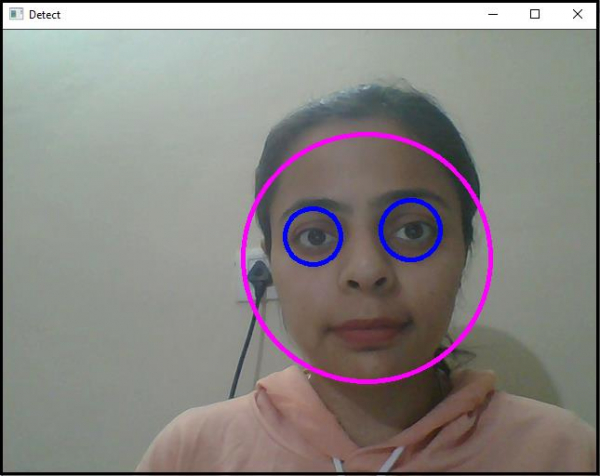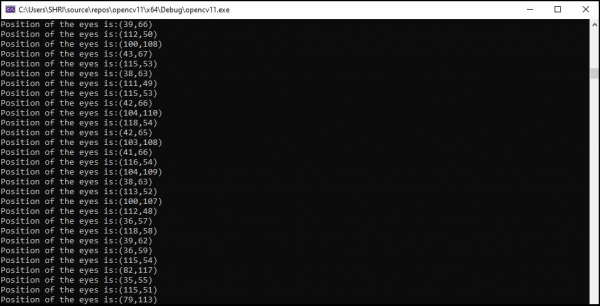# How to track the eye in OpenCV using C++?

Here, we will learn how to track the eye in OpenCV. After detective the eyes, the tracking is an effortless and straightforward task. We used the circle to enclose the detected eyes. Tracking the center of the circle means tracking the center of eyes. To track the center of the circle, we need two integer variables. This has been done on the first two lines (9th and 10th line) inside the main() function. The name of the integer variables is 'x_axis' and 'y_axis'.

In line 42 and 43, the horizontal and vertical coordinate values of the center have been copied to the 'x_axis' and 'y_axis' variable and these have the center of the circle. On 44th line, using 'cout' statement, we have shown the value of the center. This is how we can track the location of the eye.

The following code to track eye position in OpenCV using C++.

## Example

#include<iostream>
#include<opencv2/highgui/highgui.hpp>
#include<opencv2/imgproc/imgproc.hpp>
#include<opencv2/objdetect/objdetect.hpp>
using namespace cv;
using namespace std;
int main() {
int x_axis;//Declaring integer variables to store co-ordinate values//
int y_axis;//Declaring integer variables to store co-ordinate values//
Mat frame;//Declaring a matrix to video frame in it//
namedWindow("Detect");//Declaring a window to show our work//
VideoCapture image(0);//capturing video from default camera//
cout << "Couldn't load video from the source.Make sure your camera is working properly." << endl;
system("pause");
return 0;
}
double height = image.set(CAP_PROP_FRAME_HEIGHT, 480);//setting up height of each frame//
double width = image.set(CAP_PROP_FRAME_WIDTH, 640);//setting up width of each frame//
while (true) {
std::vector<Rect>faces;//Declaring a vector named faces//
for (int i = 0; i < faces.size(); i++){ //for locating the face
Point center(faces[i].x + faces[i].width * 0.5, faces[i].y + faces[i].height * 0.5);//getting the center of the face//
ellipse(frame, center, Size(faces[i].width * 0.5, faces[i].height * 0.5), 0, 0, 360, Scalar(255, 0, 255), 4, 8, 0);//draw an ellipse on the face//
Mat faceROI = frame(faces[i]);//Taking area of the face as Region of Interest for eyes//
std::vectoreyes;//declaring a vector named eyes//
eyes_cascade.detectMultiScale(faceROI, eyes, 1.1, 2, 0 | CASCADE_SCALE_IMAGE, Size(5, 5));//detect eyes in every face//
for (size_t j = 0; j < eyes.size(); j++){ //for locating eyes//
Point center(faces[i].x + eyes[j].x + eyes[j].width * 0.5, faces[i].y + eyes[j].y + eyes[j].height * 0.5);//getting the centers of both eyes//
int radius = cvRound((eyes[j].width + eyes[j].height) * 0.25);//declaring radius of the eye enclosing circles//
circle(frame, center, radius, Scalar(255, 0, 0), 4, 8, 0);//drawing circle around both eyes//
x_axis = eyes[j].x;//storing x axis location of eyes in x_axis//
y_axis = eyes[j].y;//storing y axis location of eyes in y_axis//
cout << "Position of the eyes is:" << "(" << x_axis << "," << y_axis << ")" << endl;//showing co-ordinate values//
}
}
imshow("Detect", frame);//showing result in window named 'Detect'//
if (waitKey(30) == 27){ //wait time for each frame is 30 milliseconds//
break;
}
}
return 0;
}

## Output